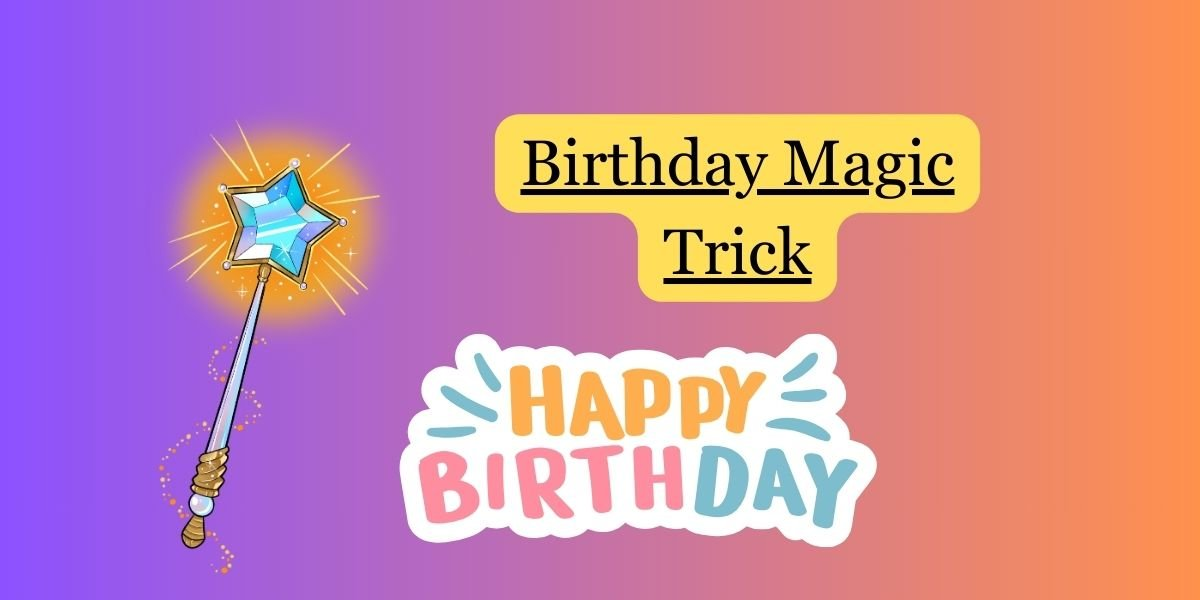Steps:

1. Ask someone to think of their birth month but not reveal it to you.
2. Instruct them to perform the following calculations mentally:
• Multiply their birth month by 5.
• Add 6 to the result.
• Multiply the result by 4.
• Add 9 to the result.
• Multiply the result by 5 again.
• Ask them to tell you the final result.
• Subtract 165 from the final result.
• Ask them to tell you the new result.
4. Reveal the magic:
• The two digits of the result represent their birth month!

Example:

• Let’s say the person’s final result is 225. If they subtract 165, the new result is 60. The digits 6 and 0 represent June, which is their birth month.

Explanation: This trick works because the series of operations involves carefully chosen numbers that result in a final number uniquely associated with their birth month.

Feel free to use this trick as a playful and surprising way to guess someone’s birth month. Just make sure they follow the steps correctly for the magic to work!